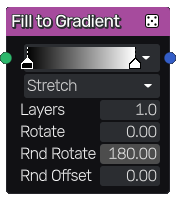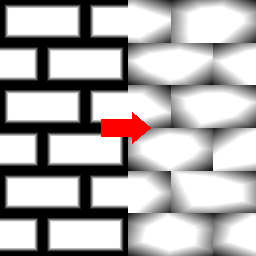The Fill to Gradient node uses the output of the Fill node and fills all detected areas with one or multiple layers of gradients.## Inputs¶

The Fill to Gradient node accepts the output of a Fill node (or a compatible output of another node) as input.

## Parameters¶

The Fill to Gradient node accepts the following parameters:

• The Mode defines how the gradient is spread out on each area (strectch or square).

• Layers defines how many layers of the gradient will be applied to each fill area, blended with the min function (darken).

• Rotate defines the base rotation of the gradient.

• Random Rotation defines how much the rotation should be randomized for each gradient.

• Random Offset defines how much each gradient get’s randomly offset.

## Example images¶